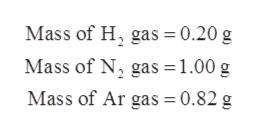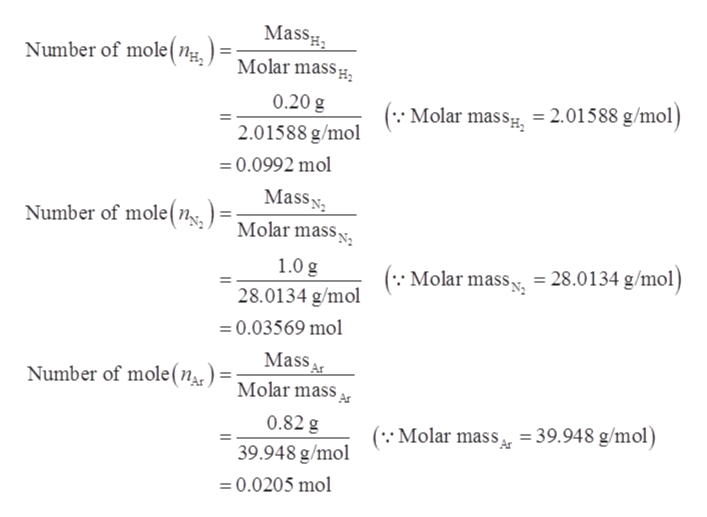# A mixture of .2 g H2, 1.00g N2 and .820 g of Ar is stored in a closed container at STP. Find the volume of the container assuming that the gases exhibit ideal behavior

Question
25 views

A mixture of .2 g H2, 1.00g N2 and .820 g of Ar is stored in a closed container at STP. Find the volume of the container assuming that the gases exhibit ideal behavior

check_circle

Step 1

Given data:help_outlineImage TranscriptioncloseMass of H gas = 0.20 g Mass of N2 gas 1.00 g Mass of Ar gas = 0.82 g fullscreen
Step 2

The number of moles is calculated by the formula shown below.

Step 3

The number of mole of H2, N2, and Ar is cal...help_outlineImage TranscriptioncloseMass Molar mass Number of mole ( n 0.20 g ( Molar mass 2.01588 g/mol) 2.01588 g/mol 0.0992 mol Mass Molar mass Number of mole(n 1.0 g Molar mass = 28.0134 g/mol) 28.0134 g/mol 0.03569 mol Mass Molar mass Number of mole (n 0.82 g ( Molar mass 39.948 g/mol) 39.948 g/mol 0.0205 mol fullscreen

### Want to see the full answer?

See Solution

#### Want to see this answer and more?

Solutions are written by subject experts who are available 24/7. Questions are typically answered within 1 hour.*

See Solution
*Response times may vary by subject and question.
Tagged in

### Physical Chemistry